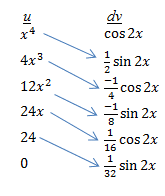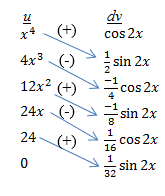Tabular integration

Occasionally, you will be faced with a complicated integral such as$\int x^4 \cos 2x \; dx$

If you’ve read some of my other posts, you know that this requires integration by parts. Let’s get started on it and see where it leads.$\begin{matrix} u = x^4 & dv = \cos 2x \; dx \\ du = 4x^3 \; dx & v = \dfrac{\sin 2x}{2} \end{matrix}$

Then (after a bit of simplifying),$\int x^4 \cos 2x \; dx = \dfrac{1}{2} x^4 \sin 2x - 2 \int x^3 \sin 2x \; dx$

Now what? Well, the new integral requires integration by parts too! So you go through all the steps again. Then you have to do integration by parts on this result. And again on the next result! It’s tedious, but eventually you get to the correct answer. However, if one of the two functions in the original integral is a polynomial, there is a faster way to do this process. It’s called tabular integration, because all the parts of the integral are found by filling in a table.

Make a two-column table, with the columns labeled u and dv. Put the polynomial function (in our example, x4) under the u. Then take the derivative of this function and write it below the original function. Continue taking the derivative until you reach 0. In our example, your first column now looks like this.$\begin{matrix} \underline{u} & \underline{dv} \\ x^4 & \text{ } \\ 4x^3 & \text{ } \\ 12x^2 & \text{ } \\ 24x & \text { } \\ 24 & \text{ } \\ 0 & \text{ } \end{matrix}$

Next, place the other function in the dv column. Integrate this function repeatedly until there are entries in every row. In our example, your completed table will look like this:$\begin{matrix} \underline{u} & \underline{dv} \\ x^4 & \cos 2x \\ \text{ } \\4x^3 & \frac{1}{2} \sin 2x \\ \text{ } \\12x^2 & \frac{-1}{4} \cos 2x \\ \text{ } \\24x & \frac{-1}{8} \sin 2x \\ \text{ } \\24 & \frac{1}{16} \cos 2x \\ \text{ } \\0 & \frac{1}{32} \sin 2x \end{matrix}$

You’ve done all the hard work! Now you can write out the integral from the values in this table. First draw an arrow from each u value (except 0) to the dv value on the next line below, as shown here:Next, place alternating plus and minus signs on each arrow:Now multiply each u value by the dv value at the other end of the arrow. The plus or minus sign tells you whether to add or subtract this term. For this problem, the integral would be:$(x^4)(\frac{1}{2} \sin 2x) - (4x^3)(\frac{-1}{4} \cos 2x) + (12x^2)(\frac{-1}{8} \sin 2x) - (24x)(\frac{1}{16} \cos 2x) + (24)(\frac{1}{32} \sin 2x)$

Simplifying gives the final answer (don’t forget the C!):$\frac{1}{2} x^4 \sin 2x \; + \; x^3 \cos 2x \; - \; \frac{3}{2} x^2 \sin 2x \; - \; \frac{3}{2} \cos 2x \; + \; \frac{3}{4} \sin 2x \; + \; C$

Blue Taste Theme created by Jabox